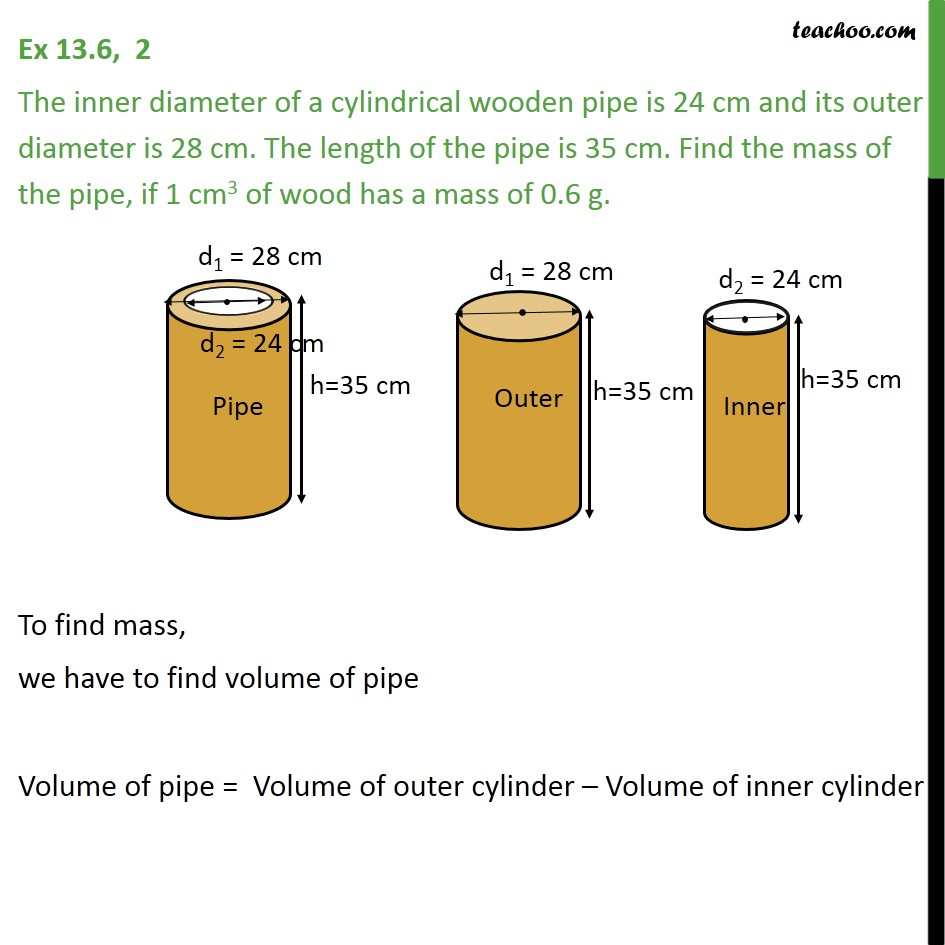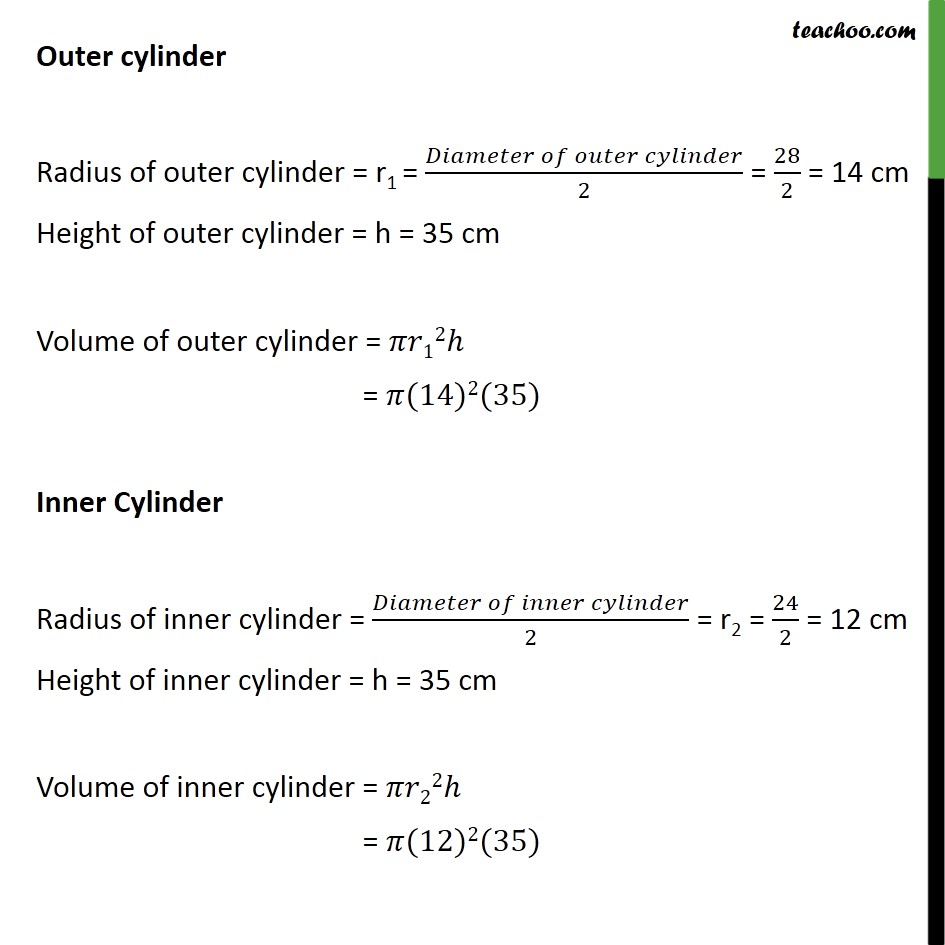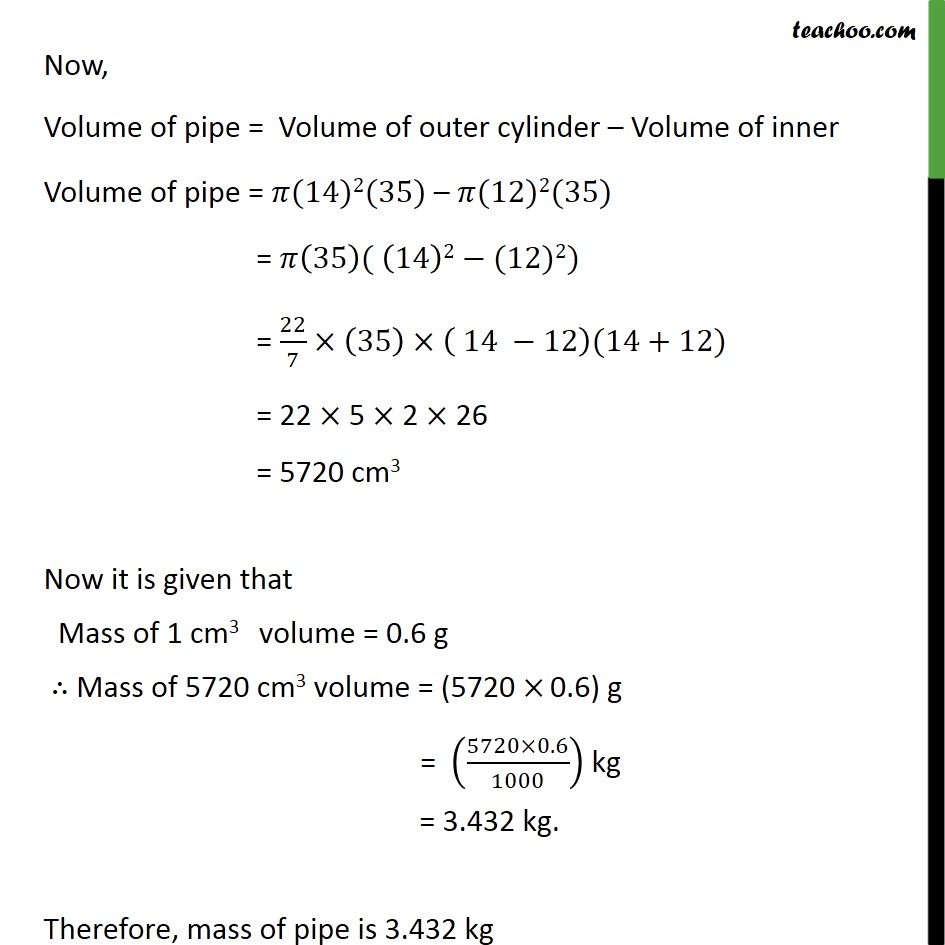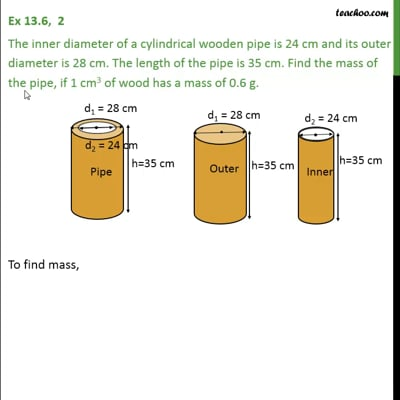Ex 13.6

Chapter 13 Class 9 Surface Areas and Volumes
Serial order wiseThis video is only available for Teachoo black users

Maths Crash Course - Live lectures + all videos + Real time Doubt solving!

### Transcript

Ex 13.6, 2 The inner diameter of a cylindrical wooden pipe is 24 cm and its outer diameter is 28 cm. The length of the pipe is 35 cm. Find the mass of the pipe, if 1 cm3 of wood has a mass of 0.6 g. To find mass, we have to find volume of pipe Volume of pipe = Volume of outer cylinder – Volume of inner cylinder Outer cylinder Radius of outer cylinder = r1 = (𝐷𝑖𝑎𝑚𝑒𝑡𝑒𝑟 𝑜𝑓 𝑜𝑢𝑡𝑒𝑟 𝑐𝑦𝑙𝑖𝑛𝑑𝑒𝑟)/2 = 28/2 = 14 cm Height of outer cylinder = h = 35 cm Volume of outer cylinder = 𝜋𝑟12ℎ = 𝜋(14)2(35) Inner Cylinder Radius of inner cylinder = (𝐷𝑖𝑎𝑚𝑒𝑡𝑒𝑟 𝑜𝑓 𝑖𝑛𝑛𝑒𝑟 𝑐𝑦𝑙𝑖𝑛𝑑𝑒𝑟)/2 = r2 = 24/2 = 12 cm Height of inner cylinder = h = 35 cm Volume of inner cylinder = 𝜋𝑟22ℎ = 𝜋(12)2(35) Now, Volume of pipe = Volume of outer cylinder – Volume of inner Volume of pipe = 𝜋(14)2(35) – 𝜋(12)2(35) = 𝜋(35)( (14)2−(12)2) = 22/7×(35)×( 14 −12)(14+12) = 22 × 5 × 2 × 26 = 5720 cm3 Now it is given that Mass of 1 cm3 volume = 0.6 g ∴ Mass of 5720 cm3 volume = (5720 × 0.6) g = ((5720×0.6)/1000) kg = 3.432 kg. Therefore, mass of pipe is 3.432 kg